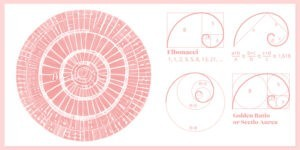Select Page

# Using Fibonacci Sequence in Layout Design

There’s more to layout design than meets the eye. Believe it or not, the Fibonacci sequence can be used to create visually interesting and appealing layouts. The Fibonacci sequence is a series of numbers in which each number is the sum of the two preceding numbers. The most famous Fibonacci sequence is 0, 1, 1, 2, 3, 5, 8, 13, 21, 34, 55, 89, 144, 233,377,610—where each number after the second is the sum of the previous two.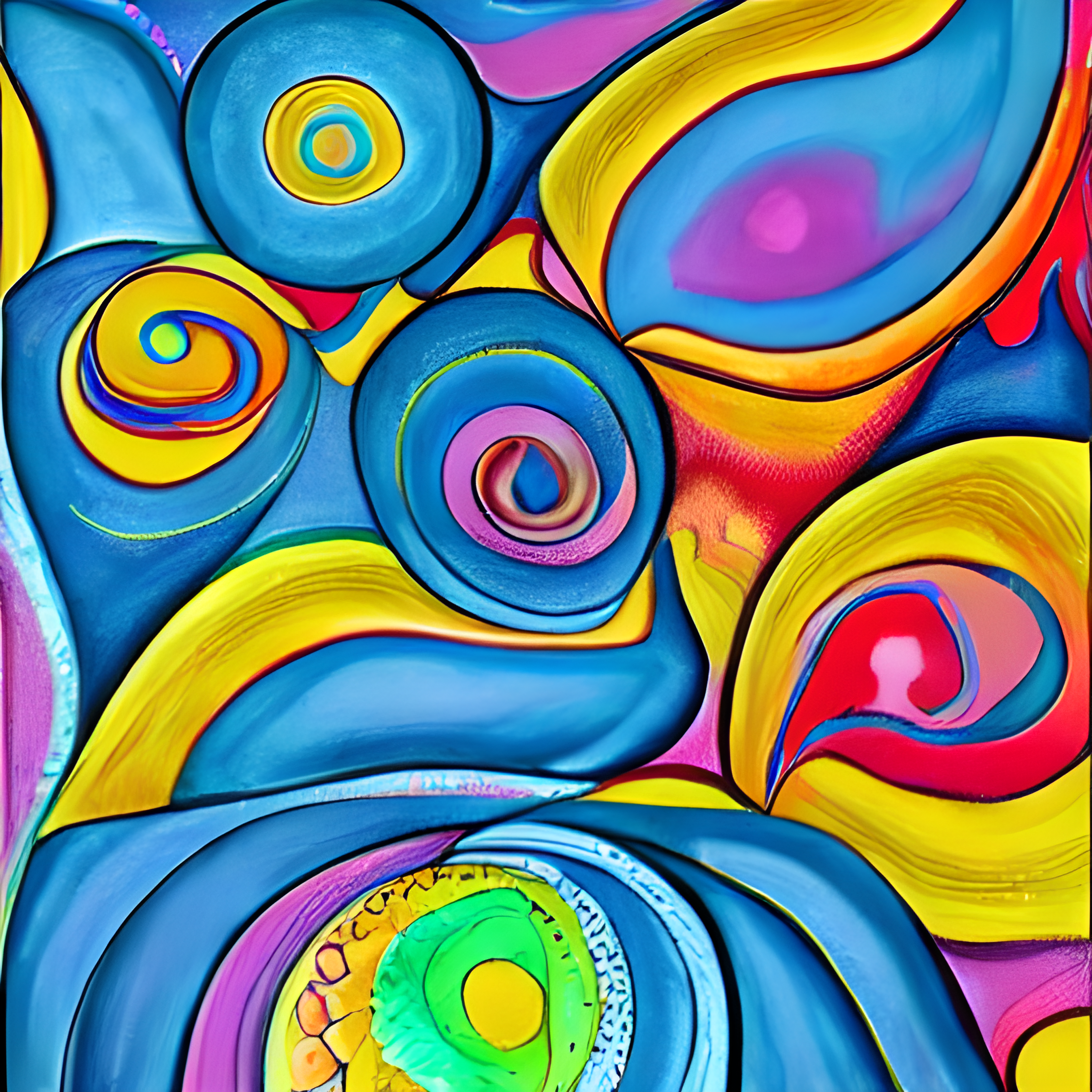modern FS art

greene edition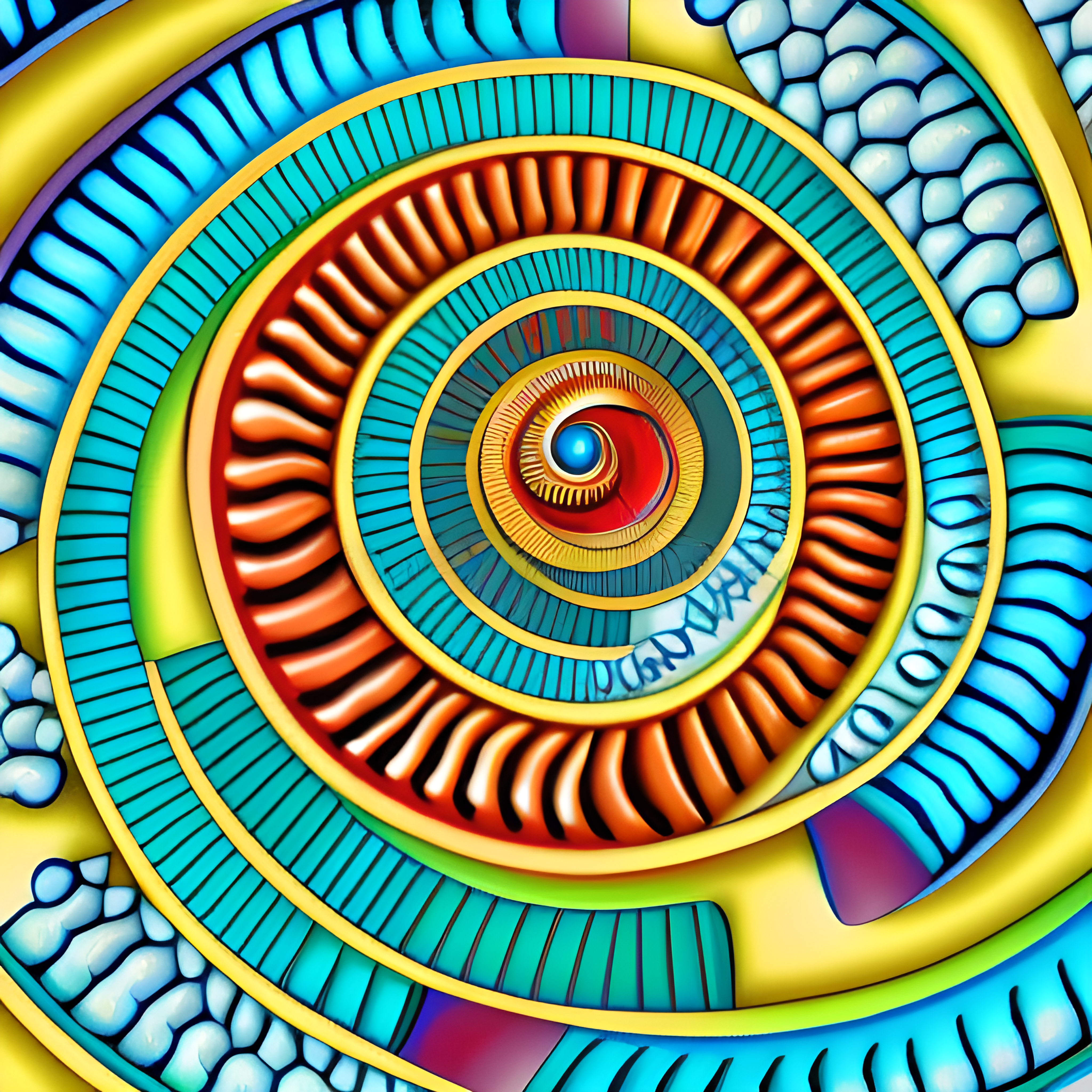# The Fibonacci Sequence: How to Use It in Layout Design

There’s more to layout design than meets the eye. Believe it or not, the Fibonacci sequence can be used to create visually interesting and appealing layouts. The Fibonacci sequence is a series of numbers in which each number is the sum of the two preceding numbers. The most famous Fibonacci sequence is 0, 1, 1, 2, 3, 5, 8, 13, 21, 34, 55, 89, 144, 233,377,610—where each number after the second is the sum of the previous two.

1. The Fibonacci sequence is a series of numbers in which each number is the sum of the two preceding numbers.

The Fibonacci sequence (FS)  is a series of numbers in which each number is the sum of the two preceding numbers. The sequence begins with 0 and 1, and each subsequent number is the sum of the previous two. The Fibonacci sequence has many applications in mathematics and other disciplines, but it can also be used in layout design. The most common use of the Fibonacci sequence in layout design is the golden rectangle, in which the length of the longer side is equal to the sum of the length of the shorter sides.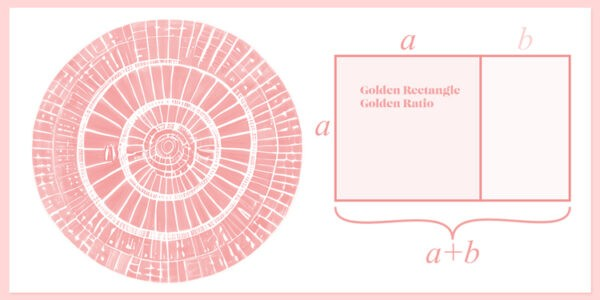The Fibonacci sequence can also be used to create Fibonacci spirals, which are visually appealing and can be used to lead the eye around a layout. To create a Fibonacci spiral, start with a square. Then, draw a quarter-circle in each corner of the square, using the length of the side of the square as the radius. Connect the endpoints of the quarter-circles to create a spiral. The Fibonacci sequence can also be used to determine the size of text and other elements on a page. For example, if you want the text on a page to be easy to read, you can use the Fibonacci sequence to determine the optimal line length.

The optimal line length is approximately 1.618 times the width of the column of text. The Fibonacci sequence can be used in a variety of other ways in layout design. Experiment with different applications to see what works best for your purposes.

## 2. The Fibonacci sequence can be used to create pleasing and balanced compositions in design.

The term “Fibonacci sequence” (FS)  is named after Leonardo of Pisa, who was also known as Fibonacci. He introduced the FS  to the Western world in his book Liber Abaci in 1202. The Fibonacci sequence is a number sequence in which each number is the sum of the previous two numbers. The sequence goes like this: 1, 1, 2, 3, 5, 8, 13, 21, 34, 55, and so on. The FS can be used in design to create pleasing and balanced compositions. Designers can use the sequence to determine the width of columns, the height of rows, and the size of margins.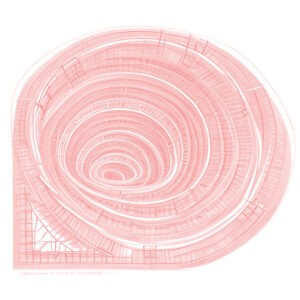The FS can also be used to create spiral compositions. To do this, designers can start with a square. They can then add a second square that is the size of the previous two squares combined. This can be repeated to create a spiral composition. The Fibonacci sequence can be used in design to create pleasing and balanced compositions. By using the sequence to determine the width of columns, the height of rows, and the size of margins, designers can create compositions that are visually appealing and have a sense of harmony.

## 3. The Fibonacci sequence is named after Italian mathematician Leonardo of Pisa, who is also known as Fibonacci.

The FS  is a series of numbers in which each number is the sum of the previous two. The sequence is named after Italian mathematician Leonardo of Pisa, who is also known as Fibonacci. The sequence can be used in layout design to create compositions that are visually appealing and balanced. For example, the Fibonacci sequence can be used to determine the width of columns in a grid.

When using the Fibonacci sequence in layout design, it is important to keep in mind the golden ratio, which is a number that is approximately 1.61. This number can be used to create compositions that are pleasing to the eye. The Fibonacci sequence is a useful tool for designers and can be used to create beautiful and balanced compositions.

## 4. The Fibonacci sequence is often visualized as a spiral

The Fibonacci spiral is a graphical representation of the FS. The sequence is often visualized as a spiral, with each number in the sequence corresponding to a point on the spiral. The Fibonacci spiral can be used to create aesthetically pleasing designs, as well as to help understand the behavior of certain mathematical functions. The FS is named after Italian mathematician Leonardo of Pisa, who is also known as Fibonacci.

The sequence was first described in his book Liber Abaci, which was published in 1202. The Fibonacci sequence is a set of numbers that starts with 0 and 1, and each subsequent number is the sum of the previous two.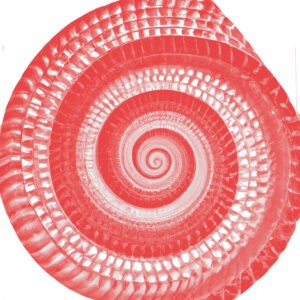The sequence can be written as: 0, 1, 1, 2, 3, 5, 8, 13, 21, 34, 55, 89, 144, 233, 377, 610, 987, 1597, 2584, 4181, 6765, 10946, 17711, 28657, 46368, 75025, 121393, 196418, 317811, 514229, 832040, 1346269, 2178309, 3524578, 5702887, 9227465, 14930352, 24157817, 39088169, 63245986, 102334155, 165580141, 267914296, 433494437, 701408733, 1134903170, 1836311903, 2971215073, 4807526976, 7778742049, 12586269025, 20365011074, 32951280099, 53316291173, 86267571272, 139583862445, 225851433717, 365435296162, 591286729879, 956722026041, 1548008755920, 2504730781961, 4052739537881, 6557470319842, 10610209857723, 17167680177565, 27777890035288, 44945570212853, 72723460248141, 11805916207174, 193039249070955, 317811305609060, 514229808101651.

As can be seen, the Fibonacci sequence is an infinite set of numbers. The ratio of consecutive Fibonacci numbers converges to a value of approximately 1.61803398874989, which is known as the golden ratio. The golden ratio has been used in art and architecture for centuries, and can be seen in works such as the Parthenon and the Mona Lisa. The FS can be used to create aesthetically pleasing designs. The golden ratio is often used in composition, as it is considered to be aesthetically pleasing. The Fibonacci spiral can be used to create a similar effect.

## 5. The Fibonacci sequence can be used in design by dividing a composition into squares or rectangles, with each rectangle having dimensions that are a Fibonacci number.

The Fibonacci sequence has been used for centuries in everything from art to music to mathematics. In design, the Fibonacci sequence can be used to create pleasing and balanced compositions by dividing a design into squares or rectangles, with each rectangle having dimensions that are a Fibonacci number. For example, if we take a square and divide it into two equal rectangles, we have a 1:2 Fibonacci ratio. Or, if we take a rectangle and divide it into a square and a smaller rectangle, we have a 2:1 Fibonacci ratio.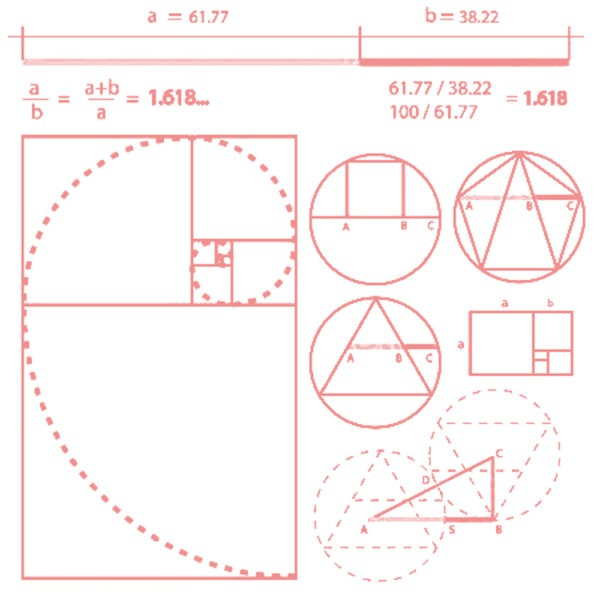Dividing a composition into squares or rectangles using Fibonacci ratios creates a pleasing, balanced design. To use the Fibonacci sequence in design, start by dividing your composition into squares or rectangles. Then, choose dimensions for each rectangle that are Fibonacci numbers. For example, you could use dimensions of 1, 1, 2, 3, 5, 8, 13, 21, 34, 55, 89, 144, etc. This will create a pleasing, balanced composition.

## 6. The Fibonacci sequence can also be used to determine the width of gutters between columns in a grid, or the space between elements in a design.

The FS can be used to determine the width of gutters between columns in a grid, or the space between elements in a design. The sequence begins with two numbers, 0 and 1, and each subsequent number is the sum of the previous two. The Fibonacci sequence can be used to create a grid system in which the width of each column is in proportion to the other columns. To do this, simply divide the width of the page by the Fibonacci sequence.

For example, if the width of the page is 1200px, the first column would be 1200px/1, the second column would be 1200px/2, the third column would be 1200px/3, and so on. This system can be used to create responsive designs that are maintain their proportions as the width of the screen changes.

The FS can also be used to determine the width of gutters between columns in a grid. To do this, simply multiply the width of the column by the Fibonacci sequence. For example, if the width of the column is 1200px, the gutter between the first and second column would be 1200px*1, the gutter between the second and third column would be 1200px*2, and so on. This system can be used to create gutters that are proportional to the width of the columns. The FS can also be used to determine the space between elements in a design.

To do this, simply add the Fibonacci sequence. For example, if the width of the element is 1200px, the space between the first and second element would be 1200px+1, the space between the second and third element would be 1200px+2, and so on. This system can be used to create space between elements that is proportional to the width of the elements.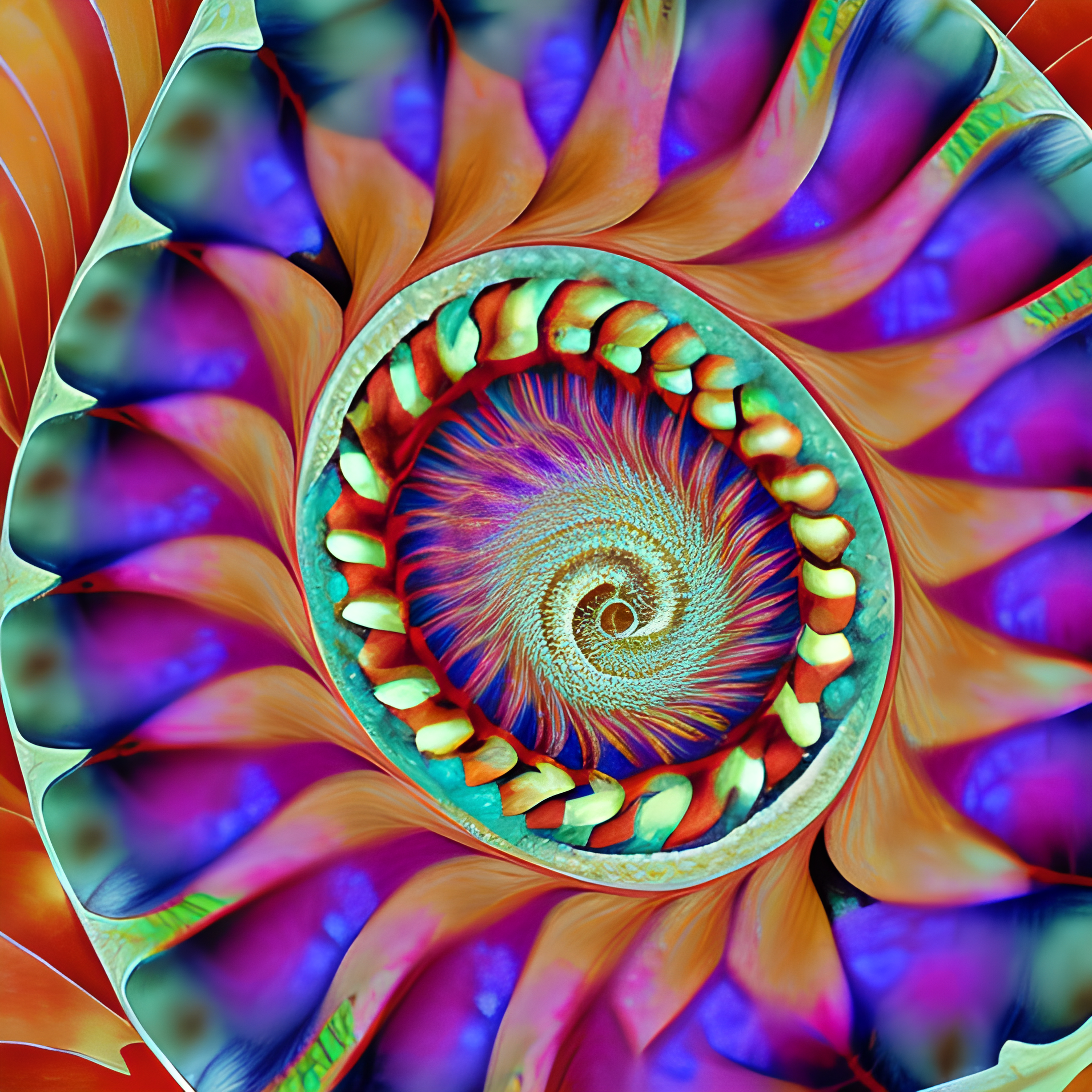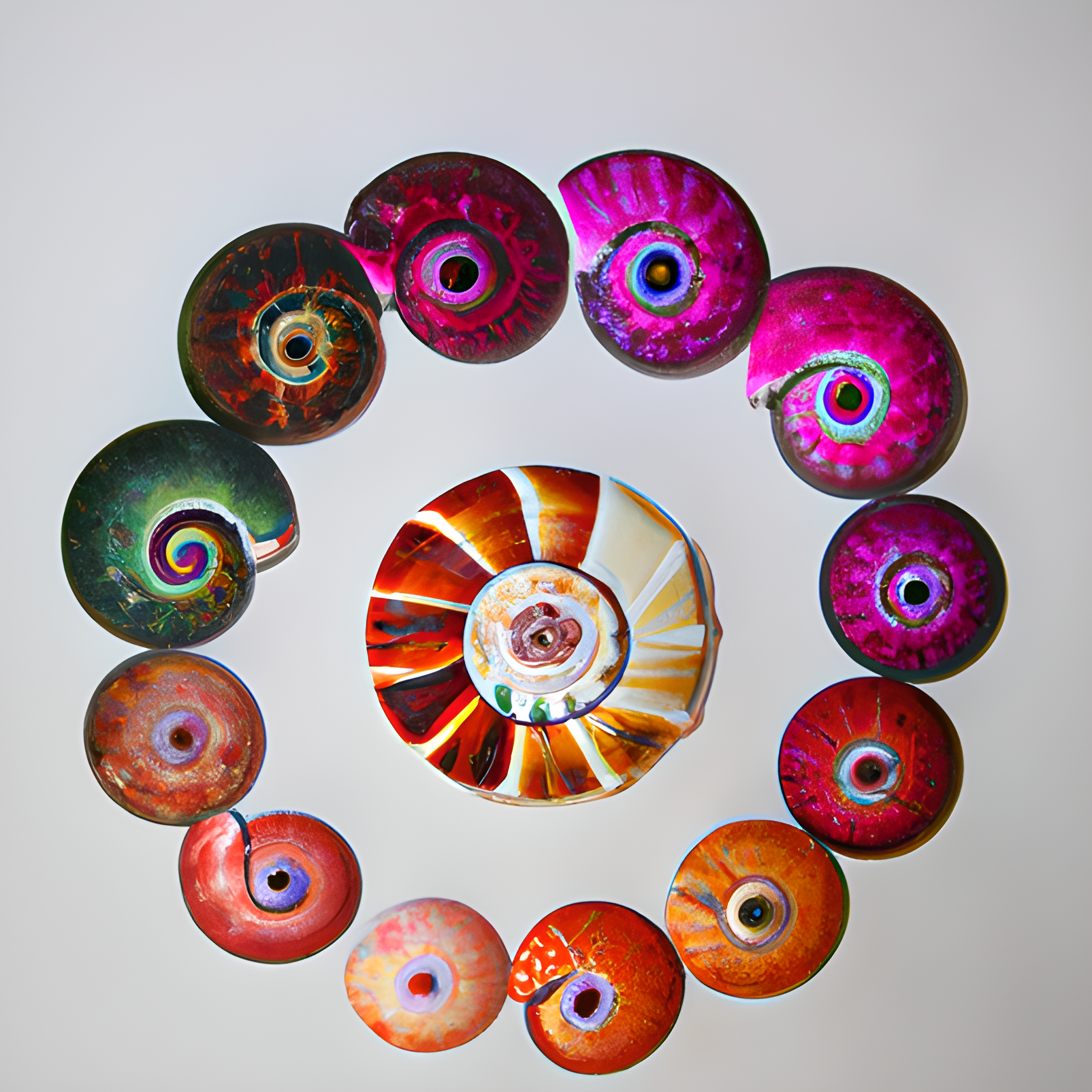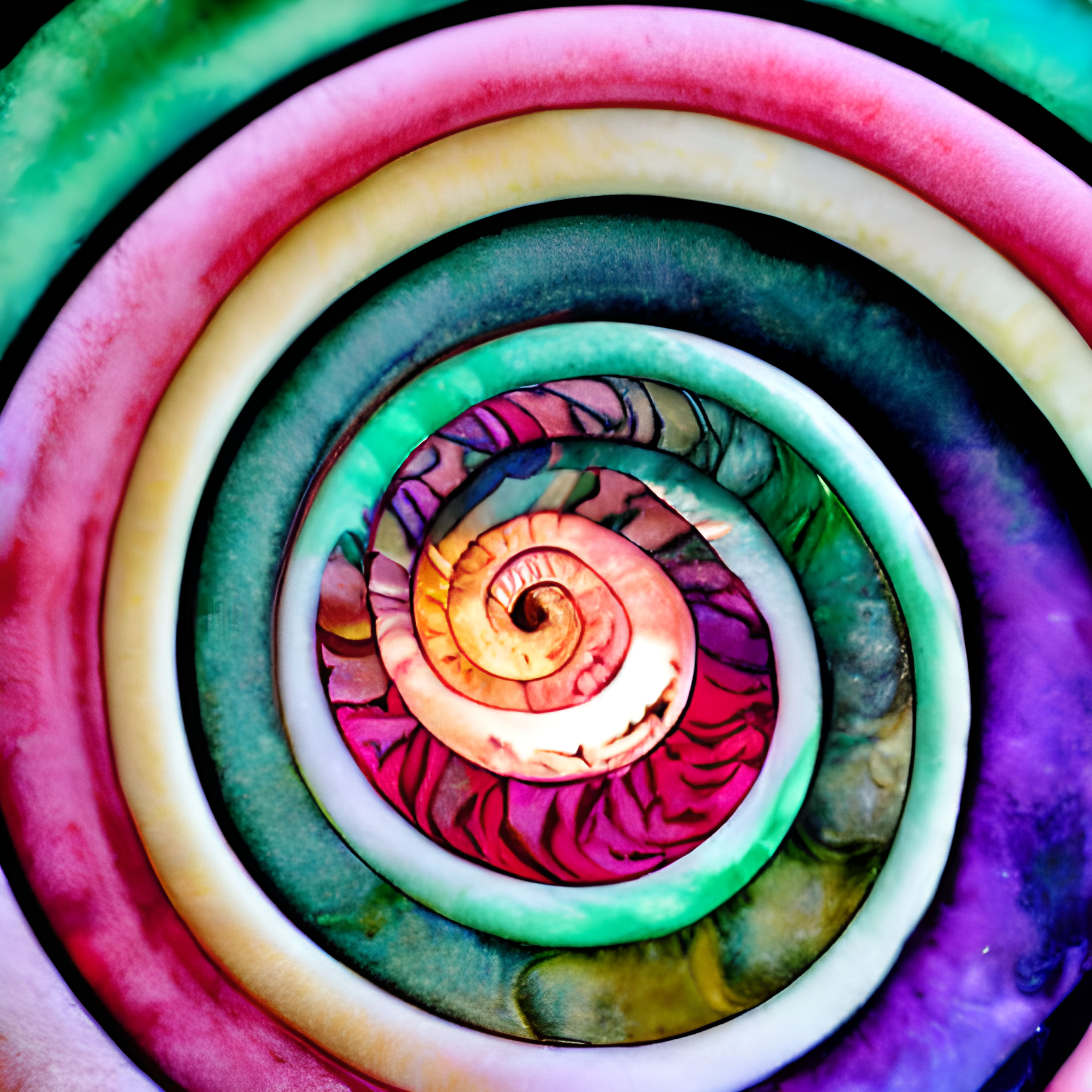## 7. Using the Fibonacci sequence in design can help create compositions that are pleasing to the eye and that feel balanced.

Balance. When designing anything, be it a website, a painting, or even just a simple layout, it’s important to create a composition that is pleasing to the eye. This can be a tricky task, but using the Fibonacci sequence can help.  The Fibonacci sequence is a series of numbers where each number is the sum of the previous two. It looks like this: 1, 1, 2, 3, 5, 8, 13, 21, 34, 55, 89, 144, etc. You can see that the pattern here is that each number is approximately 1.618 times the size of the previous number.

This ratio, called the Golden Ratio, is found throughout nature and is believed to be visually pleasing to humans. So, it makes sense that using it in design would create compositions that are also pleasing to look at. There are a few ways to use the Fibonacci sequence in design. One is to use it as a guide for sizing elements. For example, if you have two elements next to each other, you could make the larger element 1.618 times the size of the smaller element.

Or, if you have three elements, you could make the middle element the size of the sum of the other two. Another way to use the Fibonacci sequence in design is to use it as a guide for spacing elements. For example, if you have two elements, you could space them so that the distance between them is equal to the size of the larger element.

Or, if you have three elements, you could space them so that the distance between the outer two is equal to the size of the middle element. using the Fibonacci sequence in design can help create compositions that are pleasing to the eye and that feel balanced.

Few things in nature are as mathematically perfect as the Fibonacci sequence. And, it turns out, this sequence can also be used to create visually appealing design layouts. By following a few simple guidelines, you can use the Fibonacci sequence to bring harmony and balance to your design.

So next time you’re stuck on how to lay out your design, remember the Fibonacci sequence – it just might be the perfect solution.

5th anniversary tutorial: Journaling Made Easy See all greene edition anniversary tutorials here